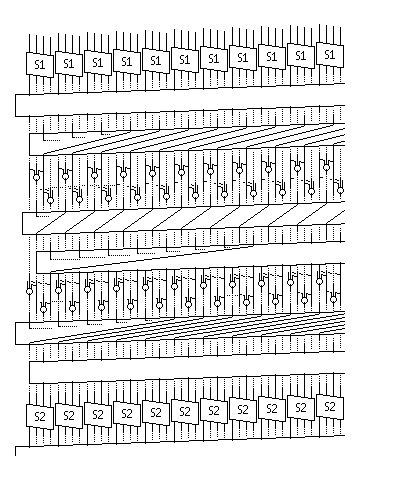[Next] [Up] [Previous] [Index]

# SERPENT

Developed by Eli Biham, the originator of differential cryptanalysis, SERPENT is a block-cipher algorithm which, like SAFER, is a "straight-through" algorithm, rather than using Feistel rounds. The original form of its description is available at http://www.cs.technion.ac.il/~biham/Reports/Serpent/. It is simple in appearance, using plain 4-bit-wide S-boxes without additional inputs (like GOST) and standard computer logic operations. It also includes an initial permutation and an inverse initial permutation, so that the S-boxes can be implemented with logic operations instead of table lookups; this is possible because the eight S-boxes used by the algorithm are used in sequence rather than in parallel.

Considering the 128 bit block as consisting of bits 0 to 127, from left to right, but with bit 0 the least significant, the first operation performed is the initial permutation. The sources of the bits resulting from that permutation are, in order, as follows:

```   0   4   8   12   16 ... 124
1   5   9   13   17 ... 125
2   6  10   14   18 ... 126
3   7  11   15   19 ... 127
```

SERPENT has 32 rounds. The last one has a slightly different sequence of operations than the other rounds.

In a normal round, the first step is to XOR the current round's subkey with the 128-bit-wide block.

Then, the entire block is transformed, nybble by nybble, according to the current S-box for the round. The S-boxes are numbered from S0 to S8, and are used in order repeatedly; S0 for rounds 1, 9, 17, and 25; S1 for rounds 2, 10, 18, and 26; and so on.

Then, the block goes through a series of mixing operations so that the different nybbles of the block interact. The process consists of ten steps, which can be thought of as being organized into five phases.

This process proceeds, in bitslice mode, as follows; for the normal mode described here, this series of steps must be preceded by the inverse of the initial permutation and followed by the initial permutation to be correct:

Considering the block as being divided into four 32-bit quarters, Q0, Q1, Q2, and Q3, from left to right, the operations are:

Rotate Q0 13 bits left, and rotate Q2 3 bits left.

Modify Q1 by XORing Q0 and Q2 to it. Modify Q3 by XORing Q0 shifted left 3 bits, and Q2 (left alone) to it.

Rotate Q1 1 bit left, and rotate Q3 7 bits left.

Modify Q0 by XORing Q1 and Q3 to it. Modify Q2 by XORing Q1 shifted left 7 bits, and Q3 (left alone) to it.

Rotate Q0 5 bits left, and rotate Q3 22 bits left.

In the final round, the mixing operations are omitted.

After the 32nd round, the bits are subjected to what is called in SERPENT the final permutation, which is the inverse of the initial permutation, and the sources of the bits resulting from it are as follows:

```   0  32  64  96    1  33  65  97    2  34  66  98    3  35  67  99
4  36  68 100    5  37  69 101    6  38  70 102    7  39  71 103
8  40  72 104    9  41  73 105   10  42  74 106   11  43  75 107
12  44  76 108   13  45  77 109   14  46  78 110   15  47  79 111
...
28  60  92 124   29  61  93 125   30  62  94 126   31  63  95 127
```

The following diagram may be of some help in understanding Serpent, although it doesn't show either the full width of the cipher or all its details.### The Key Schedule

128 and 192 bit keys are first transformed to 256 bit keys. All short keys are padded to 256 bits by having 000...001 appended to them at the most significant end, which is apparently on the right as things are defined here.

A 256 bit key is handled as follows: it is divided into eight 32-bit words. The first one is considered the oldest, and new words are generated as follows:

Word(n) = Word(n-1) XOR Word(n-5) XOR Word(n-8) XOR X'9E3779B9' XOR n

where the first generated word is considered to be word zero - so the 256 bit key consists of words -8 through -1.

128 words are generated. Then, these words are taken in groups of four to produce the round subkeys, by being put through the S-boxes. A different S-box is used, though, than the one which will be used during that round; the S-boxes are used in the sequence S3, S2, S1, S0, S7, S6, S5, S4, repeated four times.

[Next] [Up] [Previous] [Index]